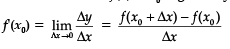The derivative of y with respect to x is th...

### Related TestThe derivative of y with respect to x is the change in y with respect to a change in x.
The derivative of f(x) at x0 is given byBased on the above data, answer the following question.
Q. Derivative of sin x with respect to x is ..........
• a)
sin x
• b)
cos xShiksha AcademyDerivative of sin x with respect to x is cos x.View courses related to this question Explore JEE courses
 Explore JEE coursesView courses related to this question1 Crore+ students have signed up on EduRev. Have you?

• ### The derivative of y with respect to x is the change in y with respect to a ... more(Scan QR code)